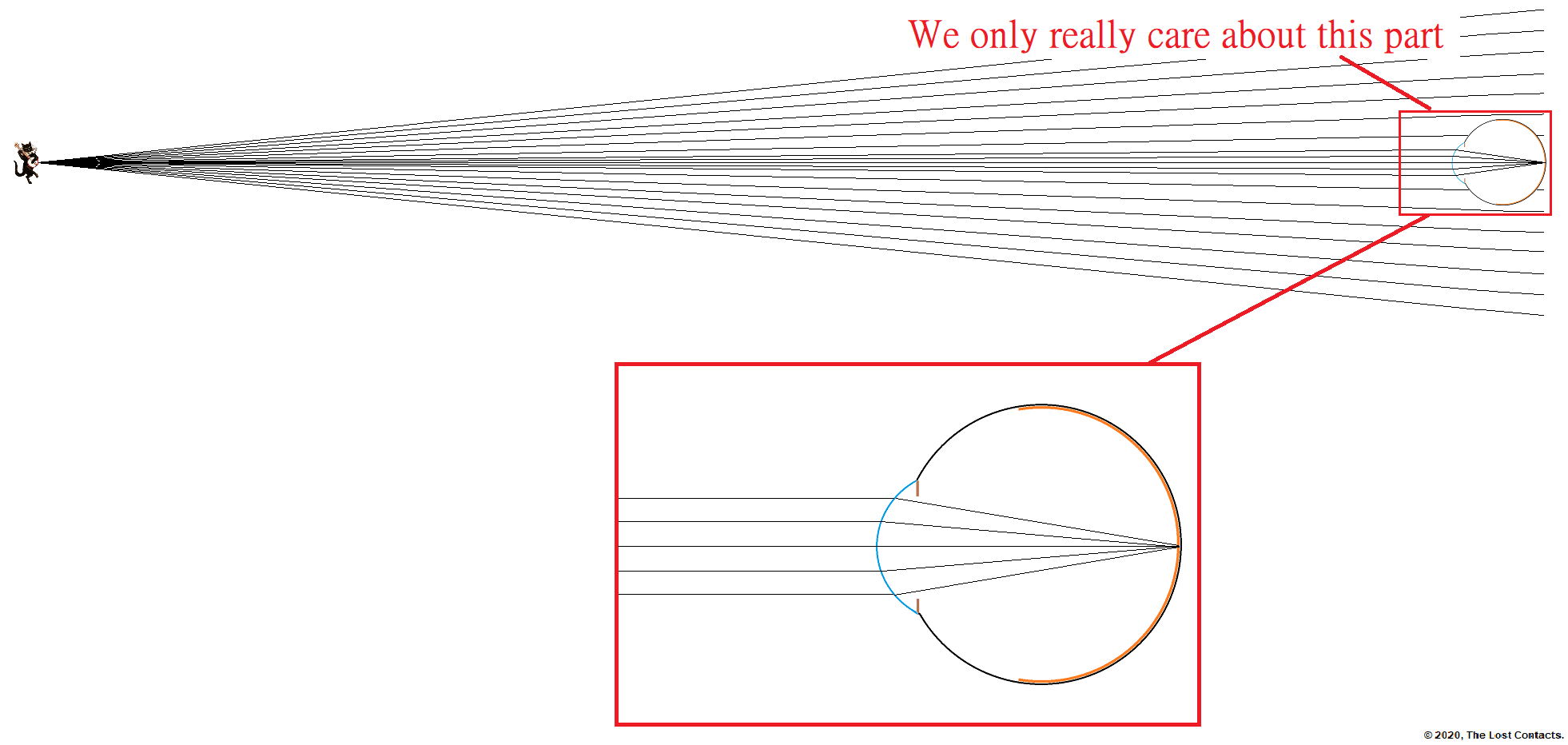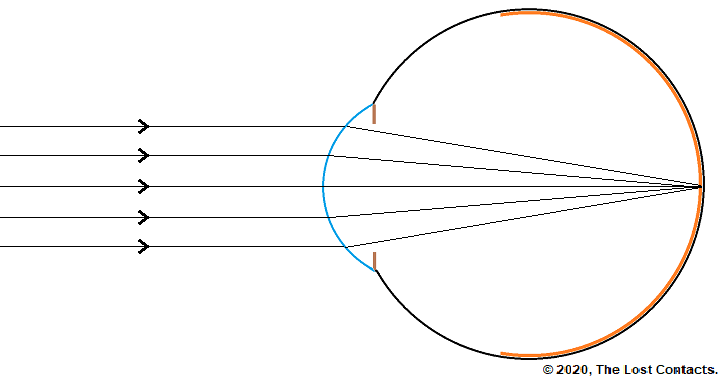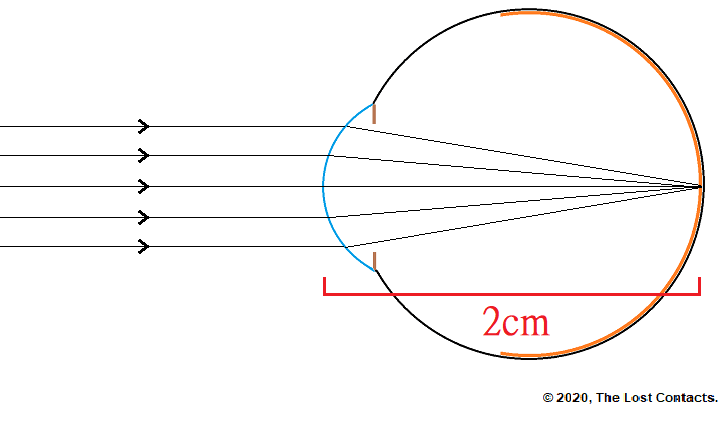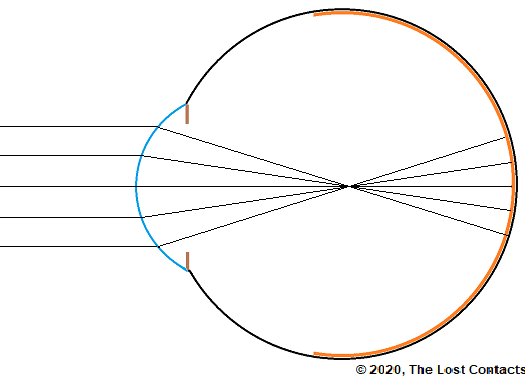In Lessons 1-4, we have built up all the knowledge needed to understand the optics and mechanics of how vision works. In this Lesson, I will be putting it all together and you will see that you haven’t been learning all of these things for nothing!

Before you proceed with this lesson, be sure to have read the following lessons:

## Object at Optical Infinity

In Lesson 1, we learned that any object can act as secondary light sources and that the light emitted by these objects is diverging. However, the divergence of the light decreases as it travels away from the source. When the light has traveled a distance 6 meters (20 feet) from the object, it has essentially lost all of its divergence. In other words, the light has become parallel. The distance at which this happens is known as optical infinity.

Remember this?When we draw ray diagrams of the eye, we often want to depict what happens when the eye is looking at objects at optical infinity. However, since we are only interested in what’s happening inside the eye itself, we will omit the object the eye is looking at and simply state that there is an object somewhere at optical infinity and only draw the incoming parallel light rays.

Including the distant object makes our diagrams needless long.Instead, we only focus on the important part and simply state or assume that there is an object out there at optical infinity.Much cleaner right? This is the type of diagram we are going to be working with from now on, and unless otherwise stated, we will assume that the eye is looking at distant objects somewhere out in optical infinity.

## Ray Diagram of The Eye

Let’s take a closer look at exactly what is happening in this diagram.## The Eye Converges Light

Here we see incoming parallel light rays that pass through the cornea and emerge as converging light rays.

Recall from Lesson 2 that plus (converging) lenses cause incoming parallel light to emerge as converging light.

Recall from Lesson 4 that the cornea is transparent and curved, which we also learned from Lesson 2 means that the cornea acts as a lens.

Therefore, if we combine these two pieces of information we can conclude that the cornea acts as a plus (converging) lens!

## The Focal Point & Focal Length

Recall from Lessons 2 & 3 that converging light eventually forms a focal point. The focal point forms a certain distance away from the plus lens (in this case it’s the cornea) depending on the power of that lens. That distance is called the focal distance or focal length.

You may now be wondering what the power of the cornea and its focal length are. I’m sure you are dying to find out!

However, due to reasons that are beyond the scope of this course, we are going to ignore a few technicalities and use a simple, made-up number for the power and the focal distance of the cornea. Don’t worry, this will not affect your understanding of the underlying principles.

## Power of the Cornea

So if we use a focal length of 2cm, can you calculate the power of the cornea?Have you figured it out?

If you’re not sure what to do, consider re-reading Lesson 3 on lens power.

If you calculated that with a focal distance of 2cm, the cornea would be +50D, not only are you correct, but you also remembered to convert from centimeters to meters! Good job!

Calculations:The focal length is 2cm.

meters = centimeters/100

In meters the focal length is 2/100 = 0.02m

The equation for lens power is:

Lens Power = 1 / Focal Length

Lens Power = 1 / 0.02m = +50D

Note: For simplicity, we will assume the cornea has a power of +50D in this and in future lessons. In reality, the cornea’s power varies from person to person and is usually between +40D and +45D.

Also note: The length of the eye is also not exactly 2cm. For most people, it is around 2.3cm, but again, this varies from person to person.

## The Retina & The Focal Point

Notice that in our diagram, the focal point is exactly on the retina. This is when the eyes can see the object they are looking at clearly.

This is extremely important to understand so I will stress it again.

The eyes see clearly when the focal point is exactly on the retina!

If the focal point were not on the retina, but rather in front or behind the retina, it would result in blurry vision.

 Example of vision when the focal point is on the retina Example of vision when the focal point is not on the retinaWe will learn more about how and why the focal point may not be exactly on the retina in Lesson 6.

** For now, the important thing to understand is that when the focal point is exactly on the retina, the vision is clear. **

And with that, let’s recap.

## Recap

• For human eyes, optical infinity is approximately 6 meters or 20 ft. The light that reaches our eyes from objects that are at or beyond optical infinity is always parallel.
• The cornea of our eyes, which acts as a plus/converging/convex lens takes that parallel light and causes it to converge.
• The converging light comes to a focal point inside the eye.
• If the focal point happens to be exactly on the retina, then our eyes can see the objects they are looking at clearly
• If for reasons we will explore in the next lesson, the focal point is not exactly on the retina, vision will be blurry.

## Summary

This lesson has combined all of the subject matter we’ve learned from previous lessons. If there is anything thus far that you don’t understand, try going back and re-reading lessons 1-4.

If you understand all of the lectures but still have questions, then it would be my pleasure to answer them. Just leave your question in the comments below!

If you don’t have any questions, then it’s time to move onto Lesson 6!